### Quantile Regression as an Optimization Problem

The model for linear quantile regression is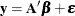where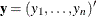is the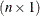vector of responses,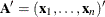is the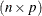regressor matrix,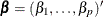is the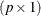vector of unknown parameters, and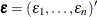is thevector of unknown errors.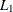regression, also known as median regression, is a natural extension of the sample median when the response is conditioned on the covariates. Inregression, the least absolute residuals estimate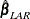, referred to as the-norm estimate, is obtained as the solution of the minimization problem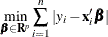More generally, for quantile regression Koenker and Bassett (1978) defined theregression quantile,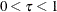, as any solution to the minimization problem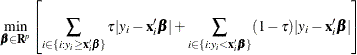The solution is denoted as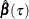, and the-norm estimate corresponds to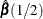. Theregression quantile is an extension of thesample quantile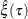, which can be formulated as the solution of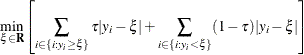If you specify weights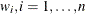, with the WEIGHT statement, weighted quantile regression is carried out by solving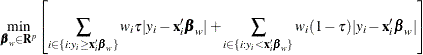Weighted regression quantiles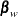can be used for L-estimation; see Koenker and Zhao (1994).